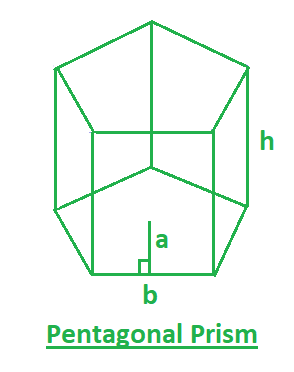Related Articles

# Program to find volume and surface area of pentagonal prism

• Last Updated : 17 Mar, 2021

A prism that has 5 rectangular faces and 2 parallel pentagonal bases is a pentagonal prism. So you are given the apothem length(a), base length(b) and height(h) of the pentagonal prism. you have to find the surface area and the volume of the Pentagonal Prism.
Examples:

Input : a=3, b=5, h=6
Output :surface area=225, volume=225

Input : a=2, b=3, h=5
Output :surface area=105, volume=75In this figure,
a– apothem length of the Pentagonal Prism.
b– base length of the Pentagonal Prism.
h– height of the Pentagonal Prism.
Formulas:Below are the formulas for calculating the surface area and the volume of the Pythagonal Prism.## C++

 // CPP program to find// surface area and volume of the// Pentagonal Prism#include using namespace std; // function for surface areafloat surfaceArea(float a, float b, float h){    return 5 * a * b + 5 * b * h;} // function for VOlumefloat volume(float b, float h){    return (5 * b * h) / 2;} // Driver functionint main(){    float a = 5;    float b = 3;    float h = 7;     cout << "surface area= " << surfaceArea(a, b, h) << ", ";     cout << "volume= " << volume(b, h);}

## Java

 // Java program to find// surface area and volume of the// Pentagonal Prismimport java.util.*; class solution{ // function for surface areastatic float surfaceArea(float a, float b, float h){     return 5 * a * b + 5 * b * h; } // function for VOlumestatic float volume(float b, float h){     return (5 * b * h) / 2; } // Driver functionpublic static void main(String arr[]){    float a = 5;    float b = 3;    float h = 7;     System.out.println( "surface area= "+surfaceArea(a, b, h)+", ");     System.out.println("volume= "+volume(b, h));}}

## Python3

 # Python 3 program to find surface area# and volume of the Pentagonal Prism # function for surface areadef surfaceArea(a, b, h):    return 5 * a * b + 5 * b * h # function for VOlumedef volume(b, h):    return (5 * b * h) / 2 # Driver Codeif __name__ == '__main__':    a = 5    b = 3    h = 7     print("surface area =", surfaceArea(a, b, h),                   ",", "volume =", volume(b, h)) # This code is contributed by# Sanjit_Prasad

## C#

 // C# program to find surface// area and volume of the// Pentagonal Prismusing System; class GFG{ // function for surface areastatic float surfaceArea(float a,                         float b, float h){    return 5 * a * b + 5 * b * h;} // function for VOlumestatic float volume(float b, float h){    return (5 * b * h) / 2;} // Driver Codepublic static void Main(){    float a = 5;    float b = 3;    float h = 7;     Console.WriteLine("surface area = " +            surfaceArea(a, b, h) + ", ");     Console.WriteLine("volume = " +                     volume(b, h));}} // This code is contributed by vt_m

## PHP

 

## Javascript

 
Output:
surface area= 180, volume= 52.5

Attention reader! Don’t stop learning now. Join the First-Step-to-DSA Course for Class 9 to 12 students , specifically designed to introduce data structures and algorithms to the class 9 to 12 students

My Personal Notes arrow_drop_up